Labib J.

Wanowrie, Pune, India - 411040# Labib J.

## Progressive classes.

Wanowrie, Pune, India - 411040.

3.9

UrbanPro Rating

Details verified of Labib J.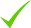IdentityEducation

Know how UrbanPro verifies Tutor details

Identity is verified based on matching the details uploaded by the Tutor with government databases.

## Overview

Labib J. describes himself as Progressive classes.. He conducts classes in Class 10 Tuition, Class 9 Tuition and Engineering Diploma Tuition. Labib is located in Wanowrie, Pune. Labib takes at students Home and Regular Classes- at his Home. He has 1 years of teaching experience . Labib is pursuing Master of Engineering - Master of Technology (M.E./M.Tech.) from Sspu. Labib has completed Bachelor of Engineering (B.E.) from Sspu in 2011. He is well versed in Marathi, Urdu, Hindi and English.

Marathi

Urdu

Hindi

English

## Education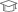Sspu Pursuing

Master of Engineering - Master of Technology (M.E./M.Tech.)Sspu 2011

Bachelor of Engineering (B.E.)

## Address

Wanowrie, Pune, India - 411040

## Verified Info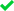Phone VerifiedEmail Verified

## Demo Class

No

Report this Profile

Is this listing inaccurate or duplicate? Any other problem?

Please tell us about the problem and we will fix it.

Please describe the problem that you see in this page.

Type the letters as shown below *

Please enter the letters as show below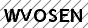## Class 9 Tuition Overview

Class 9 Tuition

Class LocationStudent's HomeTutor's Home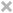Online (video chat via skype, google hangout etc)

Years of Experience in Class 9 Tuition

1

Board

State

Taught in School or College

No

State Syllabus Subjects taught

Science, Mathematics

## Reviews

this is test message this is test message this is test message this is test message this is test message this is test message this is test message

No Reviews yet! Be the first one to Review

## Answers by Labib J. (5)

Answered on 09/12/2017

Derive the formula for potential energy.

Consider body of mass is raised to a height 'h' above the ground level, the force acting on the body is the gravitational of the earth and equal to mg. This force acts in the vertically downwarddirection.To lift the body above the surface of tge earth, we will have to do work against this force of gravity. Let ... ...more
Consider body of mass is raised to a height 'h' above the ground level, the force acting on the body is the gravitational of the earth and equal to mg. This force acts in the vertically downwarddirection.To lift the body above the surface of tge earth, we will have to do work against this force of gravity. Let Work= force×displacement W = m×g×h W=mgh This work done on the body is actually the increase in potential energy of tge body. Hence work done is equal to potential energy. PE = mgh.
Answers 1 Comments
Dislike Bookmark

Answered on 08/12/2017

Mass number of Helium = 4 Number of protons = 2 Number of neutrons(n) = Number of Mass(A) Number of proton (P) = 4 -- 2 = 2 Thus, No. of neutrons = 2
Answers 9 Comments
Dislike Bookmark

Answered on 07/12/2017

Let L= length of wire d=daimeter of wire R= resistance of wire in ohms Give data L= 1m d= 0.2mm converted mm into meter d=0.2 10^-3 m R= 10? R=p L/A Area=pi (d/2)^2 =3.14 (0.2 10^-3/2)^2 =3.1415 10^-08 R=p L/A R=pL/A 10=p (1/3.1415 10^-08) Resistivity =3.14 10^-07 ?m
Answers 6 Comments
Dislike Bookmark

Answered on 07/12/2017

Mean will be 75
Answers 49 Comments
Dislike Bookmark

Answered on 07/12/2017

Let consider 141x + 103y = 217.....equation (1) 103x + 141y=27..equation(2) Multiple eq(1) by 103 we get.. 14523x + 10609y=22352..eq(3) Similarly multiple eq(2) by 141 we get.. 14523x + 19881y=3807..eq(4) Now solve eq 3 nd 4 14523x +10609y=22351 14523x+19881y=3807 - ... ...more
Let consider 141x + 103y = 217.....equation (1) 103x + 141y=27..equation(2) Multiple eq(1) by 103 we get.. 14523x + 10609y=22352..eq(3) Similarly multiple eq(2) by 141 we get.. 14523x + 19881y=3807..eq(4) Now solve eq 3 nd 4 14523x +10609y=22351 14523x+19881y=3807 - - - ____________________ -9272y= 18544 Y= -2 Put y=-2 in eq (1) 141x + 103×(-2)=217 141x - 206=217 141x=206+217 141x=423 X=3 So we get X=3 & Y=-2
Answers 16 Comments
Dislike Bookmark

Labib J.Directions

x
Class 9 Tuition 3.9

Class LocationStudent's HomeTutor's HomeOnline (video chat via skype, google hangout etc)

Years of Experience in Class 9 Tuition

1

Board

State

Taught in School or College

No

State Syllabus Subjects taught

Science, Mathematics

Class 10 Tuition 3.9

Class LocationStudent's HomeTutor's HomeOnline (video chat via skype, google hangout etc)

Years of Experience in Class 10 Tuition

1

Board

State

Taught in School or College

No

State Syllabus Subjects taught

Science, Mathematics

Engineering Diploma Tuition 3.9

Class LocationStudent's HomeTutor's HomeOnline (video chat via skype, google hangout etc)

Engineering Diploma Branch

Automobile Engineering Diploma, Mechanical Engineering Diploma

Mechanical Engineering Diploma Subject

Applied Mathematics, Fundamentals of Electronics, Theory of Machines & Mechanisms, Mechatronics, Design of Machine Elements, Industrial Fluid Power, Power Plant Engineering, Strength of Materials, Power Engineering, Mechanical Engineering Drawing, Fluid Mechanics and Machinery

Automobile Engineering Diploma Subject

Applied Math, Theory of Machines & Mechanisms, Strength of Materials, Mechanical Engineering Drawing, Automobile Transmission Systems, Automobile component Design

Type of class

Regular Classes, Crash Course

Class strength catered to

One on one/ Private Tutions

Taught in School or College

No

this is test message this is test message this is test message this is test message this is test message this is test message this is test message

No Reviews yet! Be the first one to Review

## Answers by Labib J. (5)

Answered on 09/12/2017

Derive the formula for potential energy.

Consider body of mass is raised to a height 'h' above the ground level, the force acting on the body is the gravitational of the earth and equal to mg. This force acts in the vertically downwarddirection.To lift the body above the surface of tge earth, we will have to do work against this force of gravity. Let ... ...more
Consider body of mass is raised to a height 'h' above the ground level, the force acting on the body is the gravitational of the earth and equal to mg. This force acts in the vertically downwarddirection.To lift the body above the surface of tge earth, we will have to do work against this force of gravity. Let Work= force×displacement W = m×g×h W=mgh This work done on the body is actually the increase in potential energy of tge body. Hence work done is equal to potential energy. PE = mgh.
Answers 1 Comments
Dislike Bookmark

Answered on 08/12/2017

Mass number of Helium = 4 Number of protons = 2 Number of neutrons(n) = Number of Mass(A) Number of proton (P) = 4 -- 2 = 2 Thus, No. of neutrons = 2
Answers 9 Comments
Dislike Bookmark

Answered on 07/12/2017

Let L= length of wire d=daimeter of wire R= resistance of wire in ohms Give data L= 1m d= 0.2mm converted mm into meter d=0.2 10^-3 m R= 10? R=p L/A Area=pi (d/2)^2 =3.14 (0.2 10^-3/2)^2 =3.1415 10^-08 R=p L/A R=pL/A 10=p (1/3.1415 10^-08) Resistivity =3.14 10^-07 ?m
Answers 6 Comments
Dislike Bookmark

Answered on 07/12/2017

Mean will be 75
Answers 49 Comments
Dislike Bookmark

Answered on 07/12/2017

Let consider 141x + 103y = 217.....equation (1) 103x + 141y=27..equation(2) Multiple eq(1) by 103 we get.. 14523x + 10609y=22352..eq(3) Similarly multiple eq(2) by 141 we get.. 14523x + 19881y=3807..eq(4) Now solve eq 3 nd 4 14523x +10609y=22351 14523x+19881y=3807 - ... ...more
Let consider 141x + 103y = 217.....equation (1) 103x + 141y=27..equation(2) Multiple eq(1) by 103 we get.. 14523x + 10609y=22352..eq(3) Similarly multiple eq(2) by 141 we get.. 14523x + 19881y=3807..eq(4) Now solve eq 3 nd 4 14523x +10609y=22351 14523x+19881y=3807 - - - ____________________ -9272y= 18544 Y= -2 Put y=-2 in eq (1) 141x + 103×(-2)=217 141x - 206=217 141x=206+217 141x=423 X=3 So we get X=3 & Y=-2
Answers 16 Comments
Dislike Bookmark

Labib J. describes himself as Progressive classes.. He conducts classes in Class 10 Tuition, Class 9 Tuition and Engineering Diploma Tuition. Labib is located in Wanowrie, Pune. Labib takes at students Home and Regular Classes- at his Home. He has 1 years of teaching experience . Labib is pursuing Master of Engineering - Master of Technology (M.E./M.Tech.) from Sspu. Labib has completed Bachelor of Engineering (B.E.) from Sspu in 2011. He is well versed in Marathi, Urdu, Hindi and English.

Share this Profile

Recommended Profiles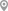Nigdi, PuneHinjewadi, PuneMoshi, PuneWanowrie, PuneWagholi, PuneWakad, Pune

X

Post your Learning Need

Let us shortlist and give the best tutors and institutes.

or

Send Enquiry to Labib J.

Let Labib J. know you are interested in their class

Reply to 's review

Enter your reply*

1500/1500

Please enter your reply

Your reply should contain a minimum of 10 charactersYour reply has been successfully submitted.

UrbanPro.com is India's largest network of most trusted tutors and institutes. Over 25 lakh students rely on UrbanPro.com, to fulfill their learning requirements across 1,000+ categories. Using UrbanPro.com, parents, and students can compare multiple Tutors and Institutes and choose the one that best suits their requirements. More than 6.5 lakh verified Tutors and Institutes are helping millions of students every day and growing their tutoring business on UrbanPro.com. Whether you are looking for a tutor to learn mathematics, a German language trainer to brush up your German language skills or an institute to upgrade your IT skills, we have got the best selection of Tutors and Training Institutes for you. Read more